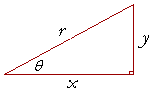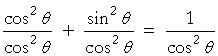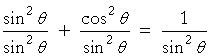# Solve Pythagorean Identities Calc#Pythagorean Identities

An online pythagorean identities solver.

sin2θ+cos2θ=1 1+tan2θ=sec2θ 1+cot2θ=csc 2θ### Pythagorean identities

 a) sin2θ + cos2θ = 1. b) 1 + tan2θ = sec2θ c) 1 + cot2θ = csc 2θ

## Proof of the Pythagorean identities

Proof 1.   According to the Pythagorean theorem,x2 + y2 = r2.  .  .  .  .  .  .  .  .  .  .  .  .  .  .  .(1)

Therefore, on dividing both sides by r2,

 x2r2 + y2r2 = r2r2 =  1.

cos2θ  +  sin2θ  =  1.  .  .  .  .  .  .  .  .  .  .  .  .  .(2)

Apart from the order of the terms, this is the first Pythagorean identity, a)

To derive b), divide line (1) by x2; to derive c), divide by y2.

Or, we can derive both b) and c) from a) by dividing it first by cos2θ and then by sin2θ.  On dividing line 2) by cos2θ, we haveThat is,

1 + tan2θ  =  sec2θ.

And if we divide a) by sin2θ, we haveThat is,

1 + cot2θ  =  csc2θ.

The 3 Pythagorean identities are thus equivalent to one another.

## Proof 2.sin2θ  + cos2θ = y2r2 + x2r2 = y2 + x2   r2 = r2r2 According to the Pythagorean theorem, = 1##Age Calculators ►

Horse Age Calculator , Cow Age Calculator , Cat Age Calculator , Dog Age Calculator, Human Age Calculator 100 Days Old Calculator Date Difference Calculator